Computational & Technology Resources
an online resource for computational,
engineering & technology publications
Civil-Comp Proceedings
ISSN 1759-3433
CCP: 83
PROCEEDINGS OF THE EIGHTH INTERNATIONAL CONFERENCE ON COMPUTATIONAL STRUCTURES TECHNOLOGY
Edited by: B.H.V. Topping, G. Montero and R. Montenegro
Paper 133

Modelling of Interaction between Reinforcement and Matrix Using the FETI Method

J. Kruis1 and P. Štemberk2

1Department of Mechanics, 2Department of Concrete Structures,
Faculty of Civil Engineering, Czech Technical University in Prague, Czech Republic

Full Bibliographic Reference for this paper
J. Kruis, P. ÂŠtemberk, "Modelling of Interaction between Reinforcement and Matrix Using the FETI Method", in B.H.V. Topping, G. Montero, R. Montenegro, (Editors), "Proceedings of the Eighth International Conference on Computational Structures Technology", Civil-Comp Press, Stirlingshire, UK, Paper 133, 2006. doi:10.4203/ccp.83.133
Keywords: fibre-matrix interaction, FETI method, bonding and debonding law.

Summary
Interaction between reinforcement and matrix is an important property which is modelled in many engineering branches. Composites with fibers or steel bars in reinforced concrete can serve as an example of the interaction.

There are several methods for description of interaction. The first method is based on special ordering of unknowns. The same displacements of points at matrix and at reinforcement have the same numbers. This method is simple but cannot be used for separation of both constituents. The second method uses different unknowns for displacements at reinforcement and matrix. Continuity conditions are enforced by a penalty method. There can be difficulty with appropriate values of the penalty parameter. A third possibility is based on interface finite elements.

This contribution deals with interaction modelled after transformation from primal variables (displacements) to dual variables (forces). The same ideas as in the FETI method are applied. The FETI method is one of the domain decomposition methods and was introduced in . The original domain is divided into several smaller subdomains which may be fixed or unconstrained. The method can deal with floating subdomains which is suitable and not common feature. Continuity conditions are enforced by Lagrange multipliers. A detailed description of the method can be found in reference .

This contribution is the first step in a series of tools for description of interaction of reinforcement and matrix. The contribution deals with interaction during initial stage of loading when no damage is assumed. Therefore perfect bonding between the reinforcement and the matrix is considered. The matrix is assumed as one domain which is supported properly. Reinforcement is modelled by floating domains which are connected with the matrix by Lagrange multipliers. The second step describes slip between the reinforcement and the matrix. A linear relationship between slip and acting force is used first and then a nonlinear constitutive law is mentioned.

Equilibrium conditions for composite matrix and reinforcement are in the form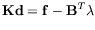(26)

where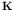denotes the stiffness matrix,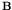denotes the signed Boolean matrix,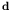denotes the vector of unknown nodal displacements and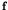denotes the prescribed nodal forces. Lagrange multipliers are collected in the vector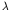. Except for the equilibrium condition, one continuity condition for perfect bonding should be used in the form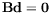(27)

The final equations are in the form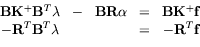(28)

which resembles the equations of the FETI method. Slip between the reinforcement and the composite matrix can be expressed in the form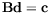(29)

which results in(30)

This contribution deals with modelling of interaction between the composite matrix and reinforcement. The presented numerical approach is based on Lagrange multipliers and the equations used resemble the equations from the FETI method. The FETI method is used as a numerical framework where several constitutive laws between reinforcement and the composite matrix can be utilized. The perfect interaction without slip leads to the classical FETI method. A linear relationship between slip and force leads to slight modification of the FETI algorithm. The general nonlinear constitutive law between slip and force leads to nonlinear equations.

References
1
C. Farhat, F.X. Roux, "A Method of Finite Element Tearing and Interconnecting and its Parallel Solution Algorithm", International Journal for Numerical Methods in Engineering, 32, 1205-1227, 1991. doi:10.1002/nme.1620320604
2
J. Kruis Domain Decomposition Methods for Distributed Computing Saxe-Coburg Publications, Kippen, Stirlingshire, United Kingdom, 2006.

purchase the full-text of this paper (price £20)